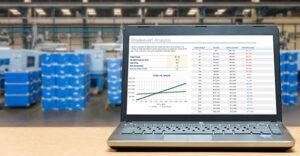#### Fixed vs. Variable Costs: How to Compute BreakevenBreakeven analysis can be useful when investing in new equipment, launching a new product or analyzing the effects of a cost reduction plan. The breakeven point is fairly easy to calculate using information from your company’s income statement. Here are the details.

Breakeven can be explained in a few different ways. It’s the point at which total sales are equal to total expenses. More specifically, it’s where net income is equal to zero and sales are equal to variable costs plus fixed costs.

To calculate your breakeven point, you need to understand a few terms:

Fixed expenses. These are the expenses that remain relatively unchanged with changes in your business volume. Examples: property taxes, salaries, insurance and depreciation.

Variable/semi-fixed expenses. Your sales volume determines the ebb and flow of these expenses. If you had no sales revenue, you’d have no variable expenses and your semifixed expenses would be lower. Examples: shipping costs, materials, supplies, advertising and training.

Applying the breakeven formula

The basic formula for calculating the breakeven point is:

Breakeven = fixed expenses / 1 – (variable expenses / sales).

Breakeven can be computed on various levels: It can be estimated for the company overall or by product line or division, as long as you have requisite sales and cost data broken down. For example, let’s suppose Division A generates \$12 million in revenue, has fixed costs of \$1 million and variable costs of \$10.8 million. Here’s how those numbers fit into the breakeven formula:

Annual breakeven = \$1 million / 1 – (\$10.8 million / \$12 million) = \$10 million

Monthly breakeven = \$10 million / 12 = \$833,333

As long as expenses stay within budget, the breakeven point will be reliable. In the example, variable expenses must remain at 90% of revenue and fixed expenses must stay at \$1 million. If either of these variables changes, the breakeven point will change.

Real-world applications

Many companies use breakeven point to set revenue goals and prepare budgets. In addition, breakeven analysis can tell you the amount of incremental sales you need to recoup an investment, such as buying a new machine or hiring a new salesperson. Alternatively, breakeven can help gauge the effects of cost reduction plans. Contact us if you have questions or need help working through the calculations.